Smartick is a fun way to learn math!Mar09

# Reducible Equations: What Are They? How Do We Solve Them?

In this post, we are going to explain reducible equations with several examples taken from Smartick’s daily sessions. We will begin with some easier examples, and work our way toward those that are more complicated.

If you need to review what an equation is, and its terms, you can take a look at this previous post from our blog.

A reducible equation is an equation that can be made simpler by ”reducing” its terms to an equivalent equation that is easier to solve.

#### Example 1

In this example, we have a reducible equation with an unknown (k) on only one side of the equation, but broken down additively (-8k – 2k).

The equation is reducible because we have to operate with these monomials in order to ”eliminate” the coefficient, by ”passing it” to the other side of the equation in the form of a quotient.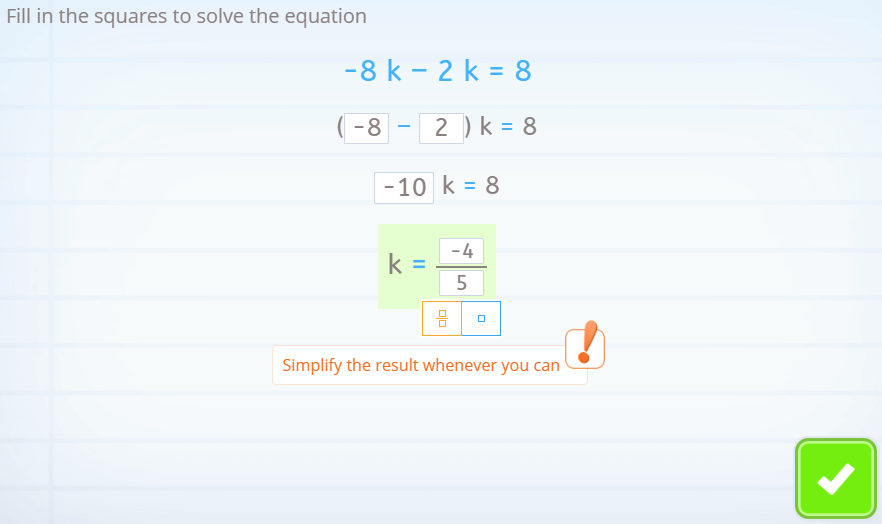#### Example 2

We are looking at a reducible equation in which the unknown (y) is only on one side of the equality, but it is related to another number additively or multiplicatively.

To isolate and resolve the unknown, we will “pass” these numbers to the other side of the equation.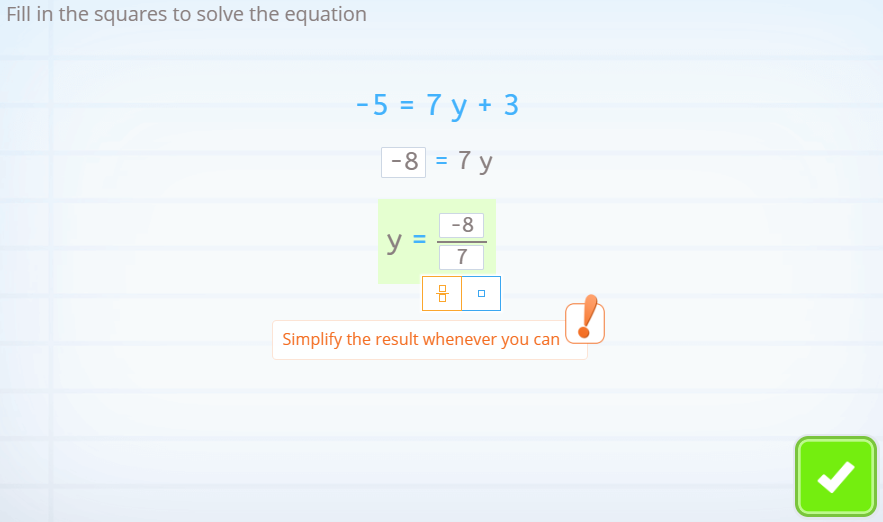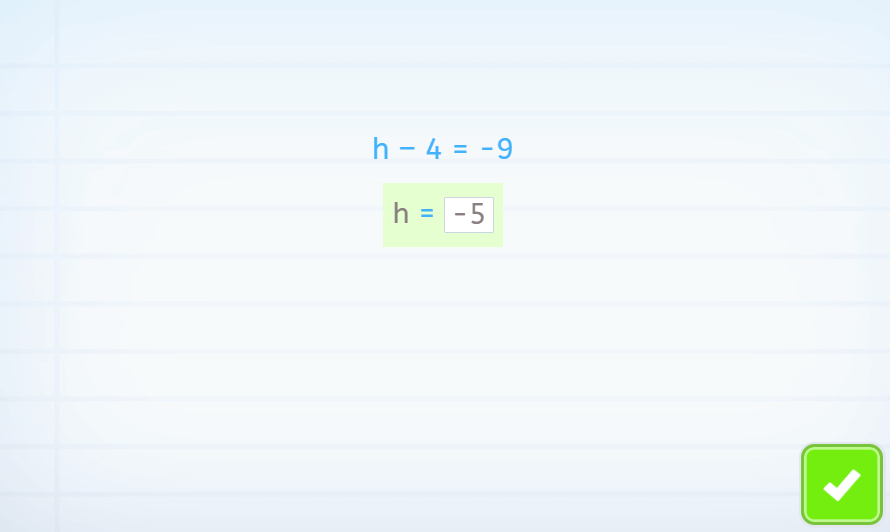#### Example 3

In this reducible equation, for the first time, we find that the unknown (t) is on both sides of the equation.

In this case, the first thing that we must do is unify all of the terms with the unknown on one side of the equation. All of the other terms must be left on the other side of the equation.

It is important to remember that all the terms are related additively, so they can be moved to the other side by adding or subtracting. Never by multiplying or dividing. This only happens when we want to move the coefficients of unknowns from one side of the equation to the other, which we have to do in the last step of these types of equations.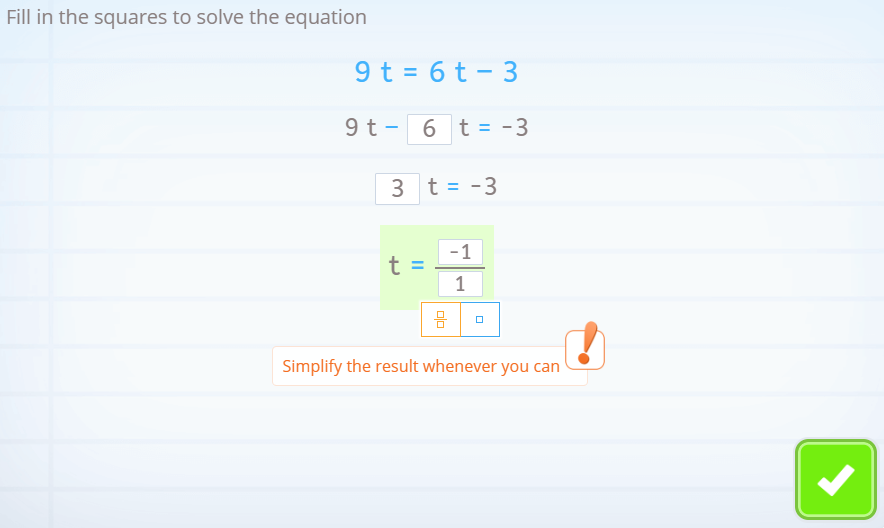#### Example 4

In this example, we find a reducible equation that presents a polynomial multiplied by a common factor.

In this case, we have to find the product and then continue as we did with the previous example. First, by changing the independent term from one side, and then by eliminating the coefficient of the unknown.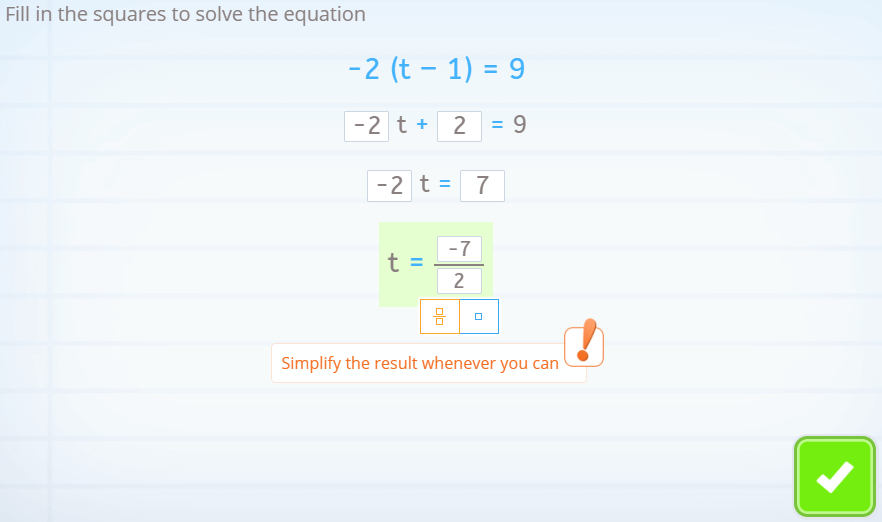#### Example 5

This is the final example that we are going to see. In this case, we start off a bit uneasy and unfamiliar with the fact that each term of the equation has a denominator.

In order to reduce this equation and create a similar, yet simpler one, we must make an equivalent equation. To do this we must multiply both terms of the equation by the same number (the least common multiple of the denominators), in order to simplify all the fractions.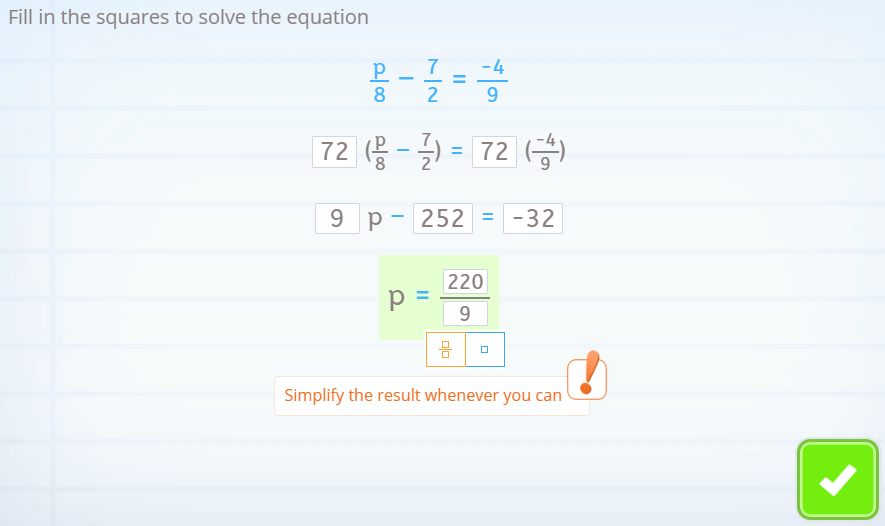I hope that with these examples you have learned what reducible equations are and how to solve them. If you would like to continue discovering math, try Smartick for free and don’t stop learning!